IceMan Reference Guide

Filtering and Convolution

Blur(x, y, blurType)
x: Width of blur in x. In pixels (Number)
y: Width of blur in y. In pixels (Number)
blurType: Type of blurring kernel (Number). Default Box

Available blur types from IceBlurType:

• Box
• Gaussian
• Bicubic

Fast, general-purpose blurring operation. Suitable for most purposes, this operation is essentially constant-time with kernel width. The different types of kernels yield different visual results. Note that each of the examples below produces a result image of a different size: as mentioned before, the rectangle occupied by a result is guaranteed to contain all non-zero contributions from the original image.

Box filtering employs an incremental moving window algorithm which makes it extremely fast. Note that IceMan allows the filter width to be non-integral, and that such widths yield the expected "weighted-sum" result.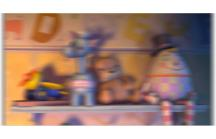blurType := IceBlurType Box result := m1_1 Blur(10, 1, blurType)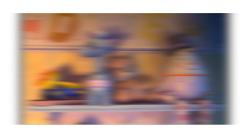blurType := IceBlurType Gaussian result := m1_1 Blur(10, 1, blurType)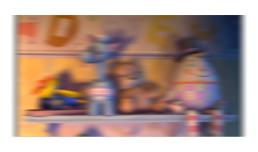blurType := IceBlurType Bicubic result := m1_1 Blur(10, 1, blurType)
Convolve(x, y, kernel)
x: Size in x of kernel (Number)
y: Size in y of kernel (Number)
kernel: Convolution kernel (List)

General convolution with arbitrary kernel. The kernel must have x * y entries.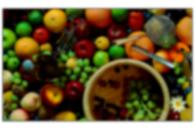// DiscKernel is a function that generates a circular convolution // kernel 30 pixels in diameter. disc := DiscKernel(30) result := fruit Convolve(30, 30, disc)

Note: This is full-fledged, no-magic-tricks convolution. For large kernels, this can be very time-consuming.

ConvolveSelective(kernelImage, pointList)
kernelImage: Image to be used as convolution kernel (IceImage)
pointList: List of points at which convolution is to be performed (List of Lists of 2 Numbers)

This is not a true convolution. Effectively, the operation is a multiplication of the kernel by the channel value at the center of the kernel, and subsequent addition with all the pixels under the kernel. This operation is performed for all points in the specified point list. Note that the points may be non-integral.

Useful for generating "sparkle" or "starburst" effects.

ConvolveTrig(kernelImage, triggerImage)
kernelImage: Image to be used as convolution kernel (IceImage)
triggerImage: "Trigger" image (IceImage)

This is not a true convolution. Effectively, the operation is a multiplication of the kernel by the channel value at the center of the kernel, and subsequent addition with all the pixels under the kernel. This operation is performed for all points in the trigger image that are not zero.

Useful for generating "sparkle" or "starburst" effects.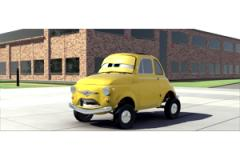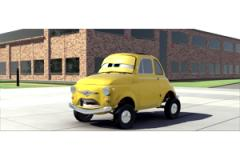// Create a starburst "kernel" sparkleType := IceSparkleType FraunhoferSlits size := List with(128, 128) center := List with(64, 64) kernel := IceImage Sparkle(sparkleType, size, center, "Fractional", 23) // Translate the kernel so that it's centered at the origin kernel := kernel Translate(-64, -64) // Perform selective convolution .. result := image ConvolveTrig(kernel, image Shuffle(0) Gt(7.9)) // É and add a fraction of the result back into the original // image result := result Add(result Multiply(0.05))

Note: The trigger image is typically generated by thresholding an input image.

Dilate(pixels)
pixels: Amount in pixels to dilate or erode (Number)

Morphological dilation/erosion operation. Positive pixel values result in dilation and negative ones in erosion. Values may be non-integral, and results vary as expected.

Note: Dilation is done by filling all values in a neighborhood with the maximum value, and erosion by filling with the minimum value. It is particularly useful for tweaking matte edges.

DirectionalBlur(width, angle, blurType)
width: Blur width in pixels (Number)
angle: Blur direction in degrees (Number)
blurType: Type of blur (Number)

Directional blur operation.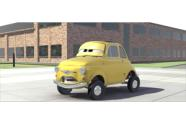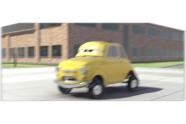blurType := IceBlurType Gaussian result = cdev DirectionalBlur(20, 20, blurType)
GaussianBlur(blurWidth)
blurWidth: Width of blur in pixels (List)

Simple Gaussian blur operation. The operation is separable, and is thus performed in one dimension at a time, but there's no other attempt at optimization. Intended for use only in special cases where Blur is not suitable.

Compute the gradient of an image using center-differencing. Each channel in the original image results in two channels in the result, corresponding respectively to the horizontal and vertical components of the gradient vector field.
Lowpass(freq)
freq: Spatial threshold frequency in x and y (List)

This operation performs convolution with a bicubic (which approximates a sinc function) such that the image is band-limited to the specified spatial frequency. The frequency is expressed as a fraction of the maximum spatial frequency representable in the image.

MedianFilter(kernelSize)
kernelSize: Size of square neighborhood in pixels (Number).

The median filter replaces all pixels in a neighborhood with the median value for that neighborhood. (Median is the value which is greater than half the pixels in the neighborhood).

Note: The median filter is sometimes useful for removing single-pixel artifacts from images, with less of a blurring effect.

Sharpen(weight, blurWidth, blurType)
weight: Sharpening "amount" (Number)
blurWidth: Width of blur kernel (Number)
blurType: Type of blurring operation used (Number)

This operation employs a technique called unsharp masking, in which the result image is a weighted sum of the original image and a blurred version of it:

result = wh.I + wl.Ib
wh = w /(2w Ð 1), wl = (1 Ð w)/(2w Ð 1)

Both blur width and type have an effect on the appearance of the final image: 5 and BLUR_GAUSSIAN are useful starting points.

VariableBlur(width, widthRange, blurType)
width: Blur width image (IceImage)
widthRange: Blur width scale (List)
blurType: Blur type (Number)

Variable-width blur operation. The extent of blur at each pixel is specified by an image. The widthRange argument specifies the blur widths corresponding to 0 and 1 in the blur-width image.